# Linearization methods

Methods that make it possible to reduce the solution of non-linear problems to a successive solution of related linear problems.

Consider a non-linear operator equation(1)

where the operatormaps a Banach spaceinto itself,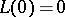, and is Fréchet differentiable. One of the classical methods for solving (1), based on linearizing (1), is the Newton–Kantorovich iterative method (cf. also Newton method; Kantorovich process), in which from a known approximation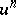a new one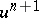is determined as the solution of the linear equation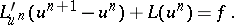(2)

The basis of the method is the approximation of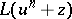(for small) by the expression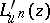, whereis the Fréchet derivative ofat the point. Various modifications of this method and corresponding estimates of the rate of convergence can be found in . The operator equation (1) itself may correspond, for example, to a non-linear boundary value problem for a partial differential equation (see , , ), and then at each stage in (2) one must solve a linear boundary value problem, which makes it necessary to use numerical methods and some discretization of the original problem and the linear problems related to it. From the computational point of view it is more natural to consider linearization methods after a suitable discretization of the original problem, taking (1) as an operator equation in a finite-dimensional space.

Another example of a linearization method for the approximate solution of (1) is the iterative method of sections (of false position) (see , ). In many cases, for problems (1) that arise in mathematical physics it is preferable to carry out the linearization ofon the basis of physical arguments, replacingby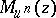for some a linear operator(see ). Then the resulting iterative methods can be written in the form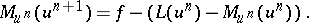(3)

Examples of methods of this kind are methods of elastic solutions and variable parameters for the solution of non-linear problems in elasticity theory (see ); for the method of elastic solutions the linear operator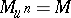corresponds to the operator of linear elasticity theory. Closely related to it are the iterative methods of Kachanov (see , ) and the method of sequential loading (see ), which combines the idea of linearization and extension with respect to the parameter. Sometimes, instead of methods such as (3) one uses more general iterative methods of the type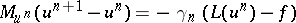(4)

with an iteration parameter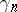subject to choice.

In the realization of these methods one should take into account the approximate nature of a solution of the systems (for example, as a consequence of the application of auxiliary iterative methods) (see , , , for example). In considering non-linear eigen value problems (problems of finding bifurcation points), for example of the form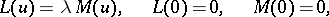(5)

the idea of linearizing (5) by reducing the investigation of the problem (5) to an investigation of the linear eigen value problemhas turned out to be very fruitful (see , ). One frequently uses linearization of one kind or other in grid methods for solving non-stationary non-linear problems (see , for example), which proceeds from known solutions at instants of time up toand gives linear equations for the solution at the next discrete instant(is the time step).